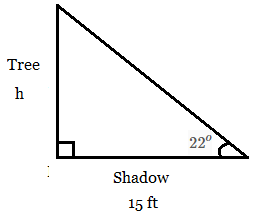# A tree casts a shadow on the ground and forms an angle of elevation of 22^o . If the shadow is...

## Question:

A tree casts a shadow on the ground and forms an angle of elevation of {eq}22^o {/eq}. If the shadow is 15 feet long, how tall is the tree?

## Trigonometric Functions:

There are 6 trigonometric ratios which are defined in terms of 3 sides (the opposite side, the adjacent side, and the hypotenuse) of a right-angled triangle. The definitions are:

$$\sin \theta = \frac{\text{opposite side}}{\text{hypotenuse}} \\ \cos \theta = \frac{\text{adjacent side}}{\text{hypotenuse}} \\ \tan \theta = \frac{\text{opposite side}}{\text{adjacent side}} \\ \csc \theta = \frac{\text{hypotenuse}}{\text{opposite side}} \\ \sec \theta = \frac{\text{hypotenuse}}{ \text{adjacent side}} \\ \cot \theta = \frac{\text{adjance side}}{\text{opposite side}}$$

We know that a tree is vertical and its shadow that is on the ground is horizontal.

The length of the shadow = 15 feet.

The angle of elevation of tree = 22 degrees.

Let us assume that {eq}h {/eq} feet is the height of the tree,

So we get:Here the side opposite to the angle {eq}22^o {/eq} is {eq}h {/eq}.

The side adjacent to the angle {eq}22^o {/eq} is 15 feet.

So we use {eq}\tan {/eq} to this angle.

$$\tan \theta = \frac{\text{opposite side}}{\text{adjacent side}} \\ \tan 22 = \dfrac{h}{15} \\ \text{Multiply both sides by 15}, \\ h= 15 \tan 22 \approx \boxed{\mathbf{6.1}} \text{ feet}$$